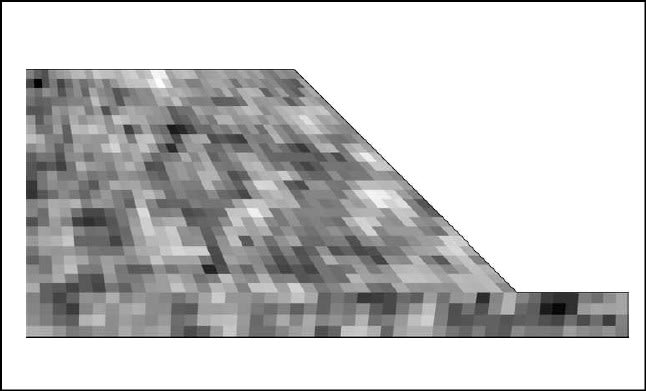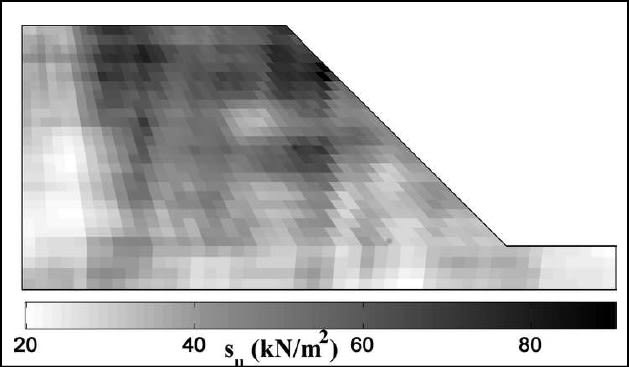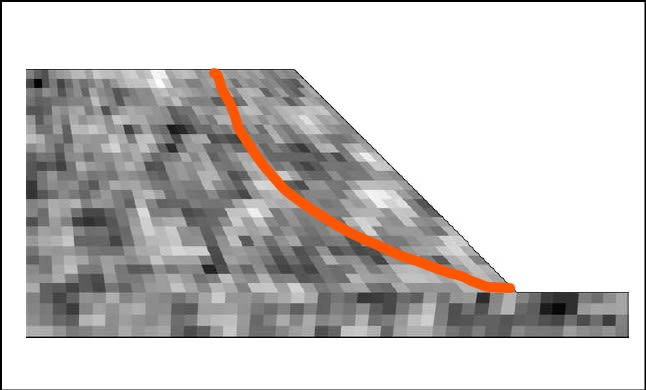×
INTELLIGENT WORK FORUMS
FOR ENGINEERING PROFESSIONALS

Are you an
Engineering professional?
Join Eng-Tips Forums!
• Talk With Other Members
• Be Notified Of Responses
• Keyword Search
Favorite Forums
• Automated Signatures
• Best Of All, It's Free!

*Eng-Tips's functionality depends on members receiving e-mail. By joining you are opting in to receive e-mail.

#### Posting Guidelines

Promoting, selling, recruiting, coursework and thesis posting is forbidden.

# Slopes and probability of failure

## Slopes and probability of failure

(OP)

The issue was basically that Pf, or Pof = Probability of failure, sometimes, or often, seems to be high in relation to the FS, the minimum Factor of safety of the slope.
One possible reason: the soil properties are known not to be constant across the volumes of interest in geotechnical engineering. They exhibit statistical variations which have been studied by the theory of the random fields, first proposed by Vanmarcke.
The scale of fluctuation or SOF describes the autocorrelation of soil properties, that is, if the fluctuations are of high or low frequency, in other words, if there are small volumes of alternating weaker and stronger soil or relatively larger volumes of alternating weaker and stronger soil.
An example of a random field model in a slope is the following, from Mohammad Tabarroki; Fauziah Ahmad; Roodabeh Banaki; Sanjay K. Jha, JGGE ASCE 2013.
The first illustration is small SOF, the second illustration is large SOF, here it is well visible that elementary soil volumes tend to cluster in weaker and stronger areas.Now, a potential slip surface will cross thru weaker and stronger fluctuations.

IF the SOF is small (figure #1), any slip surface will most probably cross thru many weaker and stronger volumes of soil, so the average strength will always govern the stability.
If the SOF is large (figure #2), the scale of the slip surface is relevant. If the scale is such that the sliding surface will cross thru weaker and larger volumes (large sliding surface relative to the dimension of the fluctuation), than the average strength governs failure.
If the dimension of the sliding surface is potentially equal to or smaller than the dimension of the fluctuation, then the surface may be randomly contained in a weaker fluctuation. In this case, it is not the average strength that governs, but rather the average strength within the weaker volumes of soil.

IN SLOPE STABILITY USUALLY THE SLIDING SURFACE IS LARGER THAN THE SCALE OF THE FLUCTUATIONS, SO THE AVERAGE STRENGTH OF THE SOIL GOVERNS.
The above means that, in probabilistic analyses, the strength parameters should be represented not by the statistical distribution of the sample, but rather by the statistical distribution of the mean of the strength parameter. This may constitute a substantial difference since often the distribution of the mean has a much lower variability than the distribution of the sample. This results in a FOS distribution with a lesser variability hence, for a given average FOS>1, a lesser probability of failure (POF), that is a lesser probability that FOS<1.

The final result, if we adopt the distribution of the sample, is often an overestimation of POF.

(to be continued)

### RE: Slopes and probability of failure

Really interesting post McCoy. By 'Standard Deviation of the means' do you mean something like: if you had 10 CPTs for a site, the correct standard deviation to use for reliability analysis would be to average the parameters from each CPT for each layer, then calculate the standard deviation of those averages (i.e. average the shear strength of the soil from 0-2m in each CPT, and then calculate the standard deviation of that average?)

### RE: Slopes and probability of failure

(OP)

#### Quote (geotechguy)

By 'Standard Deviation of the means' do you mean something like: if you had 10 CPTs for a site, the correct standard deviation to use for reliability analysis would be to average the parameters from each CPT for each layer, then calculate the standard deviation of those averages (i.e. average the shear strength of the soil from 0-2m in each CPT, and then calculate the standard deviation of that average?)

Just about that, if we simplify considering a single statistically homogeneous layer, if each CPT is considered a random sampling/§resampling through the layer, then after conversion for example from Qc to Su in your example we would have 10 values of Su average, and one corresponding values of the standard deviation of the average Su from the n=10 sample of averages.

The distribution parameters to input into the analysis software would be the average Su (often the same as the Su of all samples from all 10 CPT: the population average) and the standard of the 10 Suave. Such a standard deviation, with a sufficiently numerous dataset, is often much lower than the standard deviation of the population (that is, all the Su data extracted from all the CPTs).

It is not necessary to have a high number of in situ tests, the usual way to derive a distribution of the means is by analytical methods or by the bootstrap technique https://en.wikipedia.org/wiki/Bootstrapping_(stati...)

The analytical method is proposed by the European standard Eurocode 7, I'm going to elaborate further, the bootstrap technique can be programmed on a simple stpreadsheet.

### RE: Slopes and probability of failure

(OP)
This is a slope in a statistically homogeneous soil with a small scale of fluctuation (SOF) of its strength (described by Su or phi, according to the soil type and rupture model used). The elementary soil volumes are small and the light ones represent smaller strength whereas the darker ones represent higher strength.The overall strength across the potential sliding surface in red is given by the contribution of all the elementary volumes present across the surface. Since the surface is far larger than the scale of fluctuation, the overall strength is always governed by the average of the strength of the elementary volumes at the intersection with the surface itself.

If, in a probabilistic analysis, we use the variability of the population data, that is all the strength values of all the elementary volumes measured, we let single elementary volumes potentially govern the stability. But we know that the overall stability is the contribution of all volumes and the mean is its representative parameter. Hence, the variability of the strength to use as an input in the probabilistic analysis, in this specific case, must be the variability of the mean.

#### Red Flag This Post

Please let us know here why this post is inappropriate. Reasons such as off-topic, duplicates, flames, illegal, vulgar, or students posting their homework.

#### Red Flag Submitted

Thank you for helping keep Eng-Tips Forums free from inappropriate posts.
The Eng-Tips staff will check this out and take appropriate action.

#### Resources

Low-Volume Rapid Injection Molding With 3D Printed Molds
Learn methods and guidelines for using stereolithography (SLA) 3D printed molds in the injection molding process to lower costs and lead time. Discover how this hybrid manufacturing process enables on-demand mold fabrication to quickly produce small batches of thermoplastic parts. Download Now
Examine how the principles of DfAM upend many of the long-standing rules around manufacturability - allowing engineers and designers to place a partâ€™s function at the center of their design considerations. Download Now
Industry Perspective: Education and Metal 3D Printing
Metal 3D printing has rapidly emerged as a key technology in modern design and manufacturing, so itâ€™s critical educational institutions include it in their curricula to avoid leaving students at a disadvantage as they enter the workforce. Download Now

Close Box

# Join Eng-Tips® Today!

Join your peers on the Internet's largest technical engineering professional community.
It's easy to join and it's free.

Here's Why Members Love Eng-Tips Forums:

•Talk To Other Members
• Notification Of Responses To Questions
• Favorite Forums One Click Access
• Keyword Search Of All Posts, And More...

Register now while it's still free!